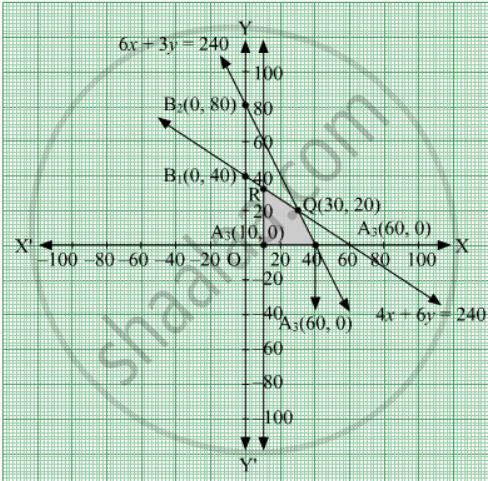Share

# Solve the Following Linear Programming Problem Graphically : Maximise Z = 7x + 10y Subject to the Constraints 4x + 6y ≤ 240 6x + 3y ≤ 240 - CBSE (Commerce) Class 12 - Mathematics

ConceptGraphical Method of Solving Linear Programming Problems

#### Question

Solve the following linear programming problem graphically :

Maximise Z = 7x + 10y subject to the constraints

4x + 6y ≤ 240

6x + 3y ≤ 240

x ≥ 10

x ≥ 0, y ≥ 0

#### Solution

The given constraints are 4x + 6y ≤ 240, 6x + 3y ≤ 240, x ≥ 10, x ≥ 0, y ≥ 0.

Firstly, convert the given inequations into equations, we obtain the following equations:

4x + 6y = 240, 6x + 3y = 240, x = 10, x = 0 and y = 0.

The line 4x + 6y = 240 meets the coordinate axes at A1(60, 0) and B1(0, 40), respectively. Join these points to obtain the line 4x + 6y = 240

Clearly (0,0) satisfies the inequation 4x + 6y ≤ 240. So, the region containing the origin represents the solution set of the inequation 4x + 6y ≤ 240.

The line 6x + 3y = 240 meets the coordinate axes at A2(40, 0) and B2(0, 80), respectively. Join these points to obtain the line 6x + 3y = 240.

Clearly (0,0) satisfies the inequation 6x + 3y ≤ 240. So, the region containing the origin represents the solution set of the inequation 6x + 3y ≤ 240.

The line x = 10 is the line that passes through A3(10, 0) and parallel to y-axis.

Region represented by x ≥ 0 and y ≥ 0:

Since, every point in the first quadrant satisfies these inequations. So, the first quadrant is the region represented by the inequations x ≥ 0 and ≥ 0.

The feasible region determined by the system of constraints is shown below:The corner points of the feasible region are A3(10, 0), A2(40, 0), Q(30, 20) and R (20, 80/3)

The values of Z at these corner points are as follows:

 Corner point Value of the objective function Z = 7x + 10y A3(10, 0) Z = 7 × 10 + 10 × 0 = 70 A2(40, 0) Z = 7 × 40 + 10 × 0 = 280 Q(30, 20) Z = 7 × 30 + 10 × 20 = 410  (Maximum) R(20, 80/3) Z = 7 xx 20 + xx 80/3 = 1220/3

Thus, the maximum value of the objective function Z is 410 which is obtained at = 30 and y = 20.

Is there an error in this question or solution?

#### Video TutorialsVIEW ALL 

Solution Solve the Following Linear Programming Problem Graphically : Maximise Z = 7x + 10y Subject to the Constraints 4x + 6y ≤ 240 6x + 3y ≤ 240 Concept: Graphical Method of Solving Linear Programming Problems.
S